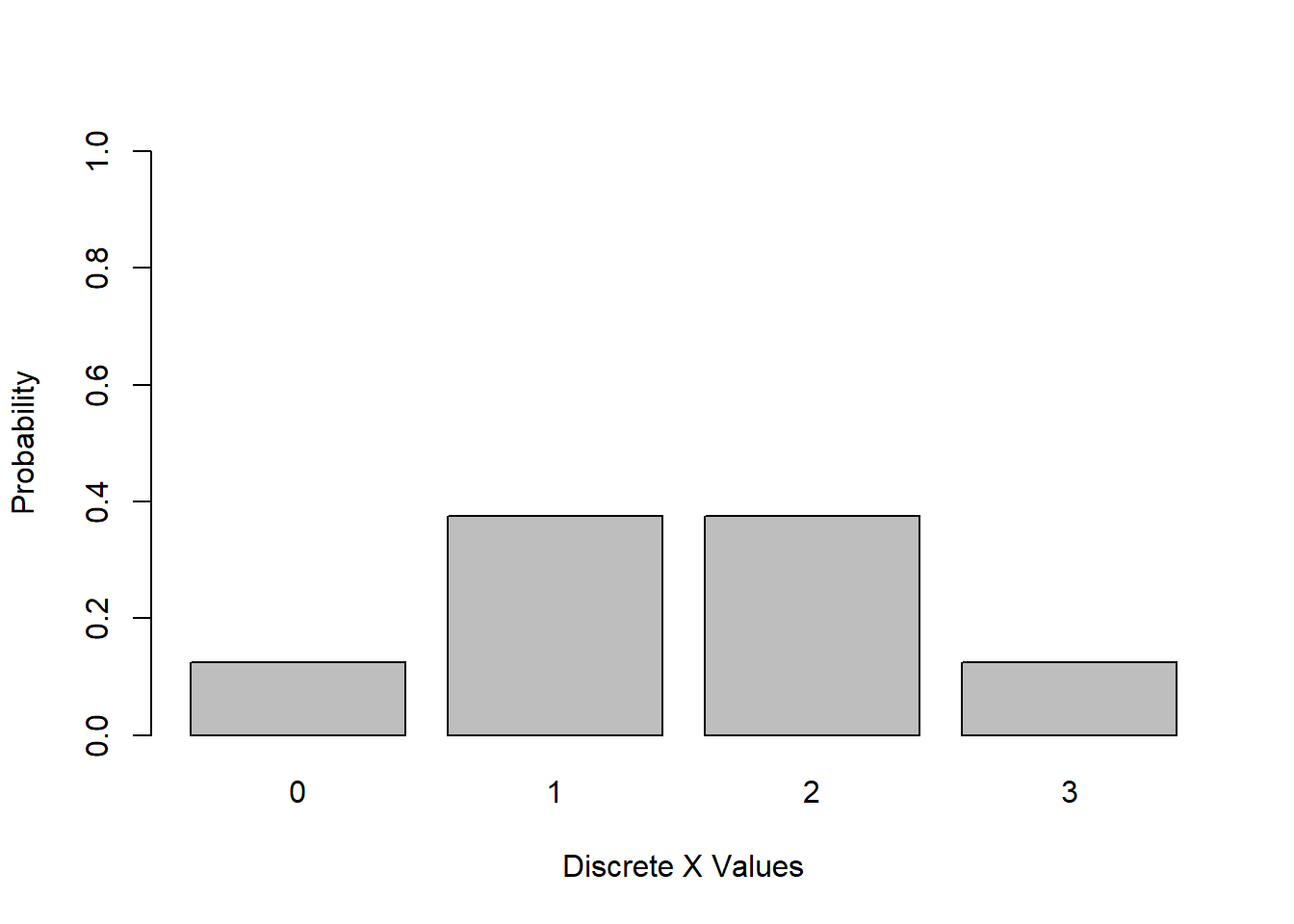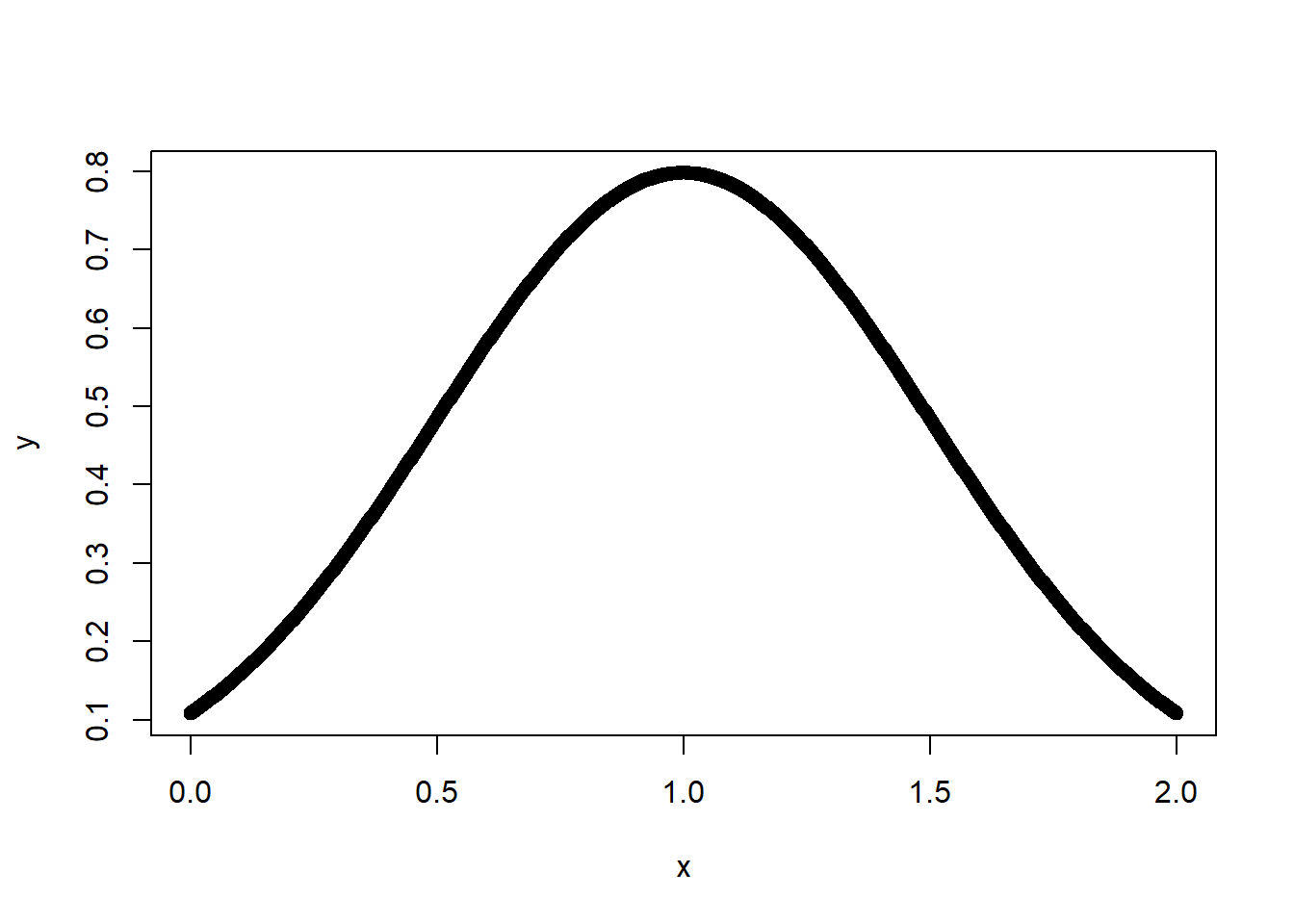# Programming Tutorials

## Discrete Vs Continuous Random Variables in R

By: Karthik Janar

## Discrete Random Variable

Random in statistics does not mean â€˜haphazardâ€™ but it means a kind of order that emerges in the long run. For example we term unpredictable things that happen in our life as â€˜randomâ€™. But we rarely see enough repetition of the same random phenomenon to observe a long term regulatrity that probability describes about â€™randomâ€™ness.

If we define X={number of heads in a three coin tosses}, then X can take ONLY values 0,1,2 and 3. Hence X is a discrete variable. And since the coin tosses resulting in Heads can occur randomly in any order, X is a DISCRETE random variable.

``````x <- c(0,1,2,3)
y <- c(1/8,3/8,3/8,1/8)
barplot(y,names.arg=x,ylab = "Probability",  xlab="Discrete X Values", ylim = c(0,1))``````## continuous Random Variable (X)

However, if we define X ={all values between 0 and 2}, there can be infinitely many possible values, so X is called a continuous random variable. This is demonstrated below.

``````x <- seq(0,2,length=1000)
y <- dnorm(x, mean = 1, sd = 0.5)
plot(x,y)``````Most Viewed Articles (in Data Science ) Latest Articles (in Data Science)

Comment on this tutorial